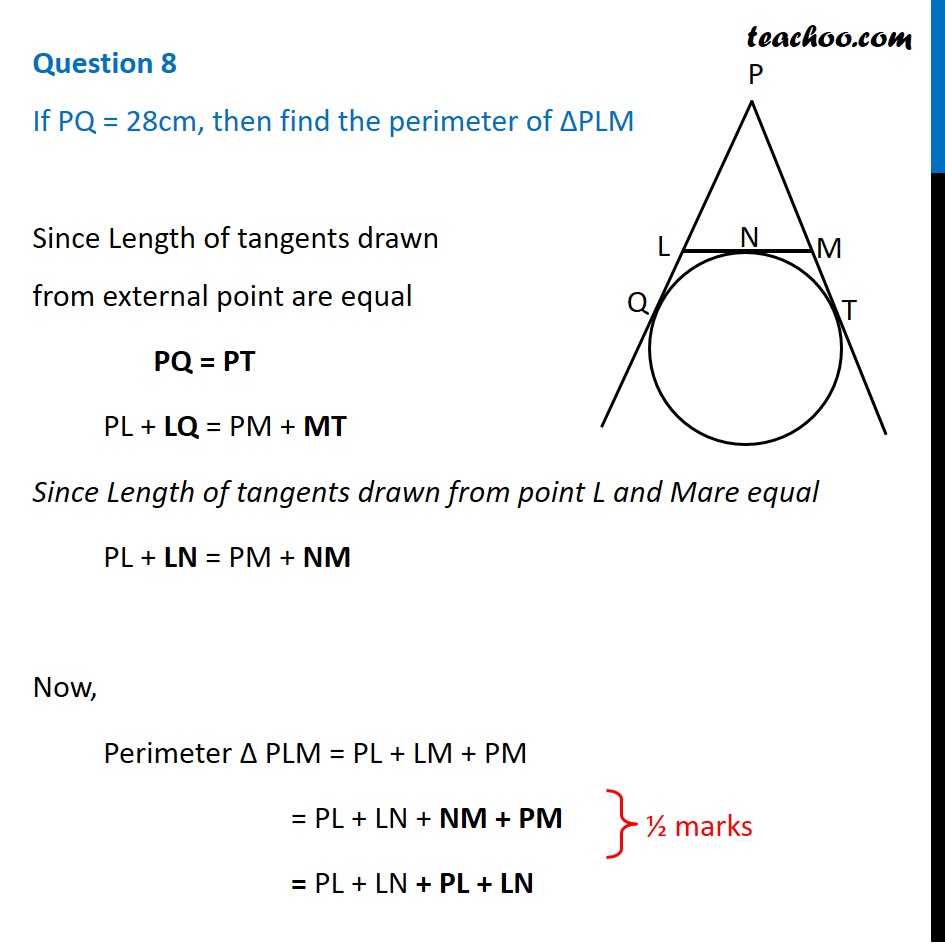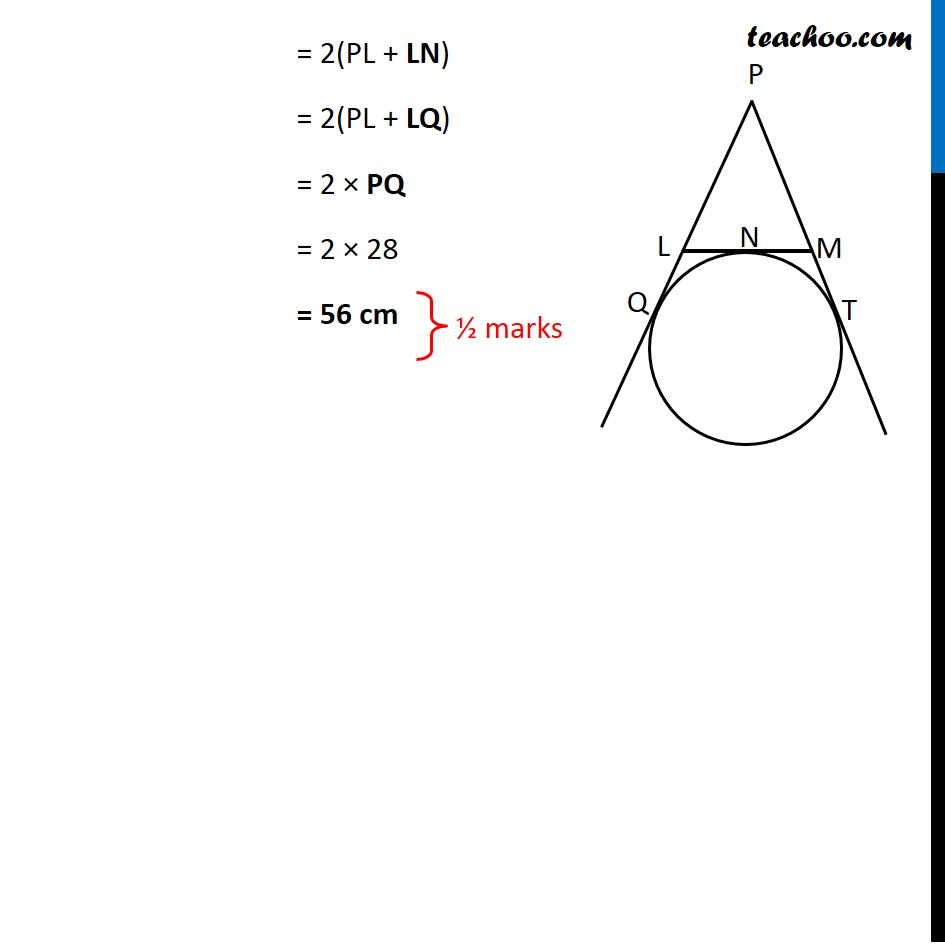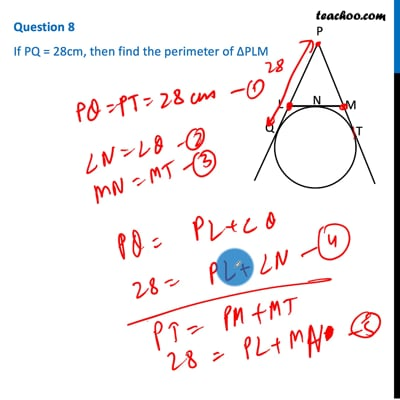CBSE Class 10 Sample Paper for 2021 Boards - Maths Standard

Class 10
Solutions of Sample Papers for Class 10 Boards

## If PQ = 28cm, then find the perimeter of ∆PLMThis video is only available for Teachoo black users

Introducing your new favourite teacher - Teachoo Black, at only ₹83 per month

### Transcript

Question 8 If PQ = 28cm, then find the perimeter of ∆PLMSince Length of tangents drawn from external point are equal PQ = PT PL + LQ = PM + MT Since Length of tangents drawn from point L and Mare equal PL + LN = PM + NM Now, Perimeter Δ PLM = PL + LM + PM = PL + LN + NM + PM = PL + LN + PL + LN = 2(PL + LN) = 2(PL + LQ) = 2 × PQ = 2 × 28 = 56 cm